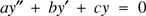Home

# Linear differential equations with constant coefficients

## Extract

We consider the second order homogeneous linear differential equation (H)

$${ ay'' + by' + cy = 0 }$$with real coefficients a, b, c, and a ≠ 0. The function y = emx is a solution if, and only if, m satisfies the auxiliary equation am2 + bm + c = 0. When the roots of this are the complex conjugates m = p ± iq, then y = e(p ± iq)x are complex solutions of (H). Nevertheless, real solutions are given by y = c1epx cos qx + c2epx sin qx.

Hide All

# Linear differential equations with constant coefficients

## Metrics

### Full text viewsFull text views reflects the number of PDF downloads, PDFs sent to Google Drive, Dropbox and Kindle and HTML full text views.

Total number of HTML views: 0
Total number of PDF views: 0 *Loading metrics...

### Abstract viewsAbstract views reflect the number of visits to the article landing page.

Total abstract views: 0 *Loading metrics...

* Views captured on Cambridge Core between <date>. This data will be updated every 24 hours.

Usage data cannot currently be displayed